AP State Syllabus AP Board 6th Class Science Important Questions Chapter 7 Let us Measure

## AP State Syllabus 6th Class Science Important Questions 7th Lesson Fun with Magnets

### 6th Class Science 7th Lesson Let us Measure 2 Mark Important Questions and Answers

Question 1.
What are conventional methods of measurement?
The conventional methods of measurement are Foot – space, Hand – space, and Cubit.

Question 2.
What are the units for length?
Meter is the standard unit of length.

Question 3.
What do you mean by area? What is the standard unit to measure area?
Square centimeter (cm2) is the standard unit to measure area.

Question 4.
What is the instrument used to measure the volume of liquids?
Measuring cylinder.

Question 5.
What is the yard?
A distance between the nose and tip of the figure is called a yard.

Question 6.
Who made the first scale? Now, where is it?
France made the first scale and now it is in France museum.

Question 7.
What was the material used by France to make the scale?
Platinum and iridium.

Question 8.
What are the instruments we used in our daily life to measure the length?
We use instruments like plane tape, roll tape, scales with different measurements made with wood plastic, and metals.

Question 9.
Give examples for having cured path?
The perimeter of the bucket and perimeter of Kadai or Tava having curved paths.

Question 10.
What is the convenient unit to measure the distance between Guntur to Visakhapatnam?
Kilo meter is a suitable unit to measure long distances.

Question 11.
Why cubit can’t be used as a standard unit of length?
The cubit can’t be used as a standard unit of length, because the length of the hand or cubit is not equal for all of the people.

Question 12.
What is a cube?
The cube is a measurement of 1 cm length 1cm breadth and 1cm of Height.

Question 13.
Which device did we use to measure the length of the curved line?
We use the thread to measure the length of a curved line.

Question 14.
How the long-distance is measured?
Long-distance is measured by kilometers.
1 kilo meter = 100 meters

Question 15.
The distance between Rama’s house and school is 2500 meters. Convert this distance in to kilometers?
1 kilometer = 1000 meters
2500 m = 2500/1000 = 2.5 km

Question 16.
What is the relation between ml and cm3?
1 ml = cm3

Question 17.
What is the unit to express the thickens of wires?
Milli meters.

Question 18.
Which instruments are used to measure the volumes of milk and liquids?
Measuring cylinders.

Question 19.
Which instruments are used to measure the plane of irregular surface?
Graph paper.

Question 20.
What is the standard unit of measuring the volume of solids?
The standard unit of measuring the volume of solids is ml.

Question 21.
Are you able to measure the volume of loose solids?
Yes, we are able to measure the volume of loose solids.

Question 22.
How can you decide a standard unit of volume of a solid?
The standard unit of volume of a solid cm3.

### 6th Class Science 7th Lesson Let us Measure 4 Mark Important Questions and Answers

Question 1.
How is the distance yard decided in the early days?

• The distance between nose and tip of the middle finger of their king was taken a measure by some people.
• They called this distance as a yard.
• This yard was divided into three equal parts and each part was called as a foot.
• They divided each foot into twelve equal parts called inches.
• Then they divided each inch into smaller parts.

Question 2.
Why there is a need to develop an internationally accepted instrument for measuring lengths?

• Each country had its own scale which differs from others, so it lead to commerce a lot of problems in trade and commerce.
• So each country in the world began to make their own sales.
• Finally in France, it was decided that a certain length of rod made of a special material (Platinum – Iridium) would be called a meter.
• This was finally accepted as an instrument of measuring lengths.

Question 3.
What is the standard unit of length? How is it divided into smaller units?

• Meter is the standard unit of length.
• The centimeter and millimeters are smaller units of length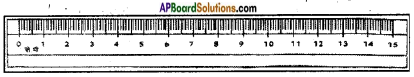• 1 Meter (1 m) = 100 centimeters (100 cm)
• 1 Centimeter (1 cm) = 10 millimeters (10 mm)

Question 4.
Describe a measuring cylinder. Where are they used?

• A measuring cylinder is a tubular structure with graduations marked on the body.
• We can fill it with liquid and can read markings at the lowest point of the concave surface of the liquid.
• Measuring cylinders are available in different sizes.
• They are used in laboratories to measure a certain volume of liquids like milk, oil etc.

Question 5.
A persons height is written as 1.85 m. Convert it in to cm and milli meters?
Person height is 1.85 m
1 meter = 100 cm
1.85 m = 1.85 x 100 = 185 cm
We know that 1 meter = 1000 mm
1.85m = 1.85 x 1000 mm = 1850 mm

Question 6.
Why meter scale is used to measure the cloth length?

• The meter scale is an internationally accepted instrument for measuring length.
• This avoids a lot of problems in international trades and commerce.
• The length measures with the meter is constant anywhere in the world.

Question 7.
Why did women use their hands to measure the jasmine flowers sting?
Accuracy is not important in measuring the jasmine string.
Various in measurement is negligible in this trade.
So, there is no problem by using hand measurement in this aspect.
And this is also an old tradition of our country that will be continued.

Question 8.
Define volume? What is its importance?
Volume is a measure of extent space that occupy a body.
Apart from the measuring of volume of liquids we also measure the volumes of solids like sand and clay.

• We use measuring cylinders to measure the volume of liquids as milk, kerosene and oil.
• The volume of liquid is expressed in liters or in ml.

Question 9.
How is meter scale designed and what is it subunits? Where it is preserved?

• In France, it was decided that a certain length of rod made of a special material (Platinum-Iridium) would be called a meter.
• The meter was divided into 100 equal parts called centimeters.
• Each centimeter was further divided into ten equal parts called millimeters.
• Now we are using this as a standard measurement for length throughout the world.
• This original scale is preserved in a museum in France.

Question 10.
How do you measure the length with a meter scale?

• The zero marks on the scale are made to coincide with one end of the table and the reading at the point which is coinciding with the other end of the table is taken.
• Since a meter scale has some thickness.
• We may make an error if the eye is not correctly positioned.
• The correct position of the eye is vertically above the end where the reading is to be taken.

Question 11.
How do you select a suitable instrument to measure length?
I select a tape to which is a suitable instrument to measure the length.

### 6th Class Science 7th Lesson Let us Measure 8 Mark Important Questions and Answers

Question 1.
What precautions must be taken while using a meter scale?
We must take the following precautions while using a meter scale: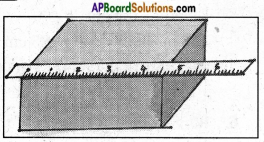• The scale should be placed exactly along length to be measured.
• Zero-point of the scale should be coincide with the starting point of the length to be measured.
• Our eyes must be vertically above the point of coincidence of scale where the measurement is to be taken.
• Ensure that the ends of scale are not worn out.
• Measure the length of an object more than two times and then take the average of these measurements for accuracy.

Question 2.
How do you measure the height of your classmate using a meter scale?

• I shall ask my classmate to stand with his / her back against a wall.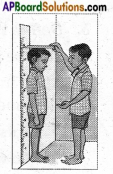• I put a mark on the wall exactly above his / her head.
• Then I measure the distance, from the floor to this mark on the wall, with a scale.
• The same procedure is followed to some other students and their observations are recorded in the table.

Question 3.
How do you measure the length accurately with a meter scale?

• In our day-to-day work, we use a wooden/plastic scale to measure lengths.
• It is marked or graduated in centimeters and millimeters.
• Take a scale. The zero mark on the scale is made to coincide with one end of the table.
• And the reading at the point which is coinciding with the other end of the table is taken.
• Since a meter-scale has some thickness, we may make an error if the eye is not correctly positioned.
• The correct position of the eye is which is vertically above the end where the reading is to be taken.

Question 4.
Observe the given of figure. In each figure, vertical and horizon¬tal lines divide the surface into certain number of parts.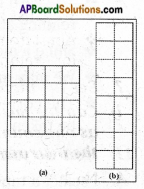i) Which figure has more area and why?
I think, both the figures have same area.

ii) Are all the parts in both figures equal?
No, in both figure length and breadth are not equal.

iii) What is the shape of the smaller part in each diagram?
It has square shape.

iv) Is the length and breadth of each smaller part are equal?
Yes, the length and breadth of each smaller part are equal.

v) Measure the length and breadth of any one part of each diagram. What do you notice?
We notice that they have equal area with different measurements.

Question 5.
How can you measure the area of a rectangular surface?

• Cut a cardboard into a shape of rectangle having length 4 cm and breadth 2 cm as shown in Figure.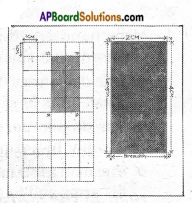• Let us measure its area. The convenient unit to measure the area of given cardboard would be cm2.
• Take a centimeter graph paper. Each small square on this graph paper has a side equal to 1 cm.
• The area of each small square on this graph paper is 1 cm2.
• Place the cardboard on the centimeter graph paper (figure).
• and draw its outline with the help of a sharp pencil.
• Now remove the cardboard and mark the shape as PQRS.
• Count the number of squares inside the outline. The number of squares is 8.
• Area of the cardboard is equal to the area covered by PQRS on the graph paper.
• Area of PQRS = Total area of unit squares inside the PQRS
= 8 x area of 1 unit square
= 8 x 1cm2 = 8 cm2
In this case, the cardboard we used has a regular shape – rectangle.

Question 6.

• Measuring cylinder is cylindrical in shape, with graduations marked on its body.
• Measuring cylinders are available in different sizes.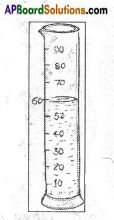• They are used in laboratories to measure a certain volume of a liquid and to measure milk, oils, etc by shop keepers.
• We can fill it with the liquid to be measured and then read the marking at the lowest point of the concave surface of liquid.
• We must bring our eyes in line with this level of liquid and then read it.
• Apart from measuring the volumes of liquids, we also measure the volumes of solids, for example, loose solids like sand, clay, and ready-mix of cement.

Question 7.
See the picture and answer the following questions?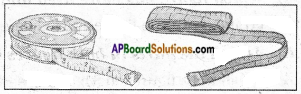i) Can we measure long distances such as one country to other country using the instruments?
No, its not possible.

ii) If not, how are these distances measured?
Long distances are used in kilo meters.

iii) What instruments are used?
An odometer is used to measure the kilometers.

iv) Is there any other way to measure very large distances?
Very long distances are generally denoted in light-years. That is, distance traveled by light in one year.

Question 8.
Narrate the story of scale?
Many hundred years ago, people used to measure distances with their hand-spans, strides or foot-spans.

• One day a very tall man went to a shop to buy some cloth. He asked for three and a half arm’s length of cloth.
• The shopkeeper measured three arm lengths of cloth and then added approximately another half-arm length.
• The man felt that the shopkeeper had cheated on him. So he measured the cloth with his arms and found that the cloth was not even three arm lengths.
• He told the shopkeeper that the length of the cloth was less than three-and-a-half arms when he measured with his own arm.
• The shopkeeper replied that his own arm was the standard for measuring. They both argued about whose arm was to be taken as a standard measure.
• In those days, people arguing over measuring the length of fields, ropes, and hundreds of other things must have been a familiar fight. How should one measure a half or a quarter arm length
• Finally, some sensible people got together and decided to have a scale of a fixed length.
• In order to measure subunits, they marked this scale with several smaller but equal divisions.
• They then decided that everyone would measure lengths with this scale.
• They used wood and metal to make scales of the same length.

Question 9.
How do you measure the volume of a cardboard box?